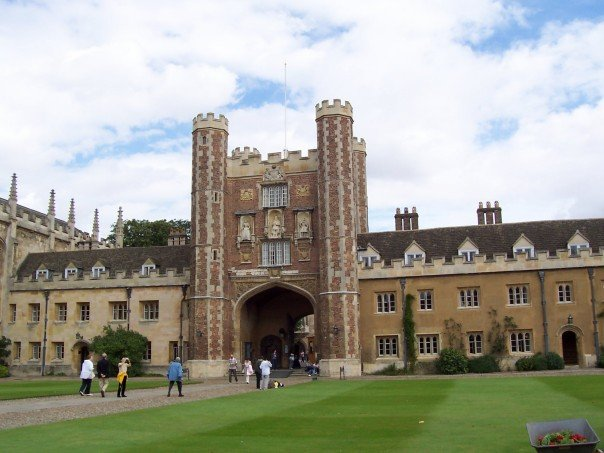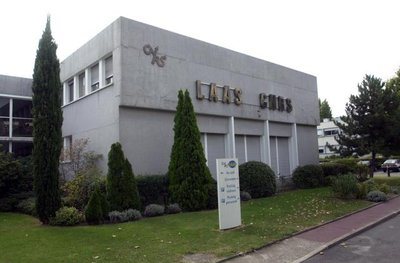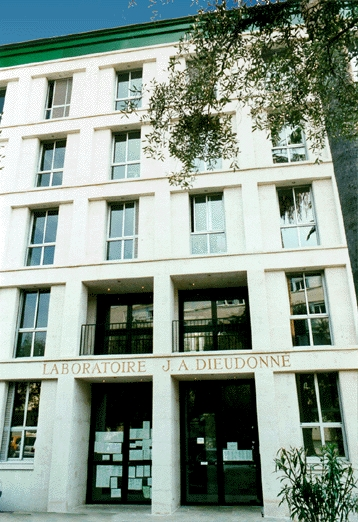[ Main Page | Research | Management | Grants | Industrial contracts | Courses | Conferences | ALEA INRIA team | Collaborators | Website on Feynman-Kac models ]

# Pierre Del Moral - Preprints## HAL, Arxiv, Cambridge University, UBC , and Imperial College of London (since 2007)## HAL-INRIA Publications (since 2007)## Preprints du L.P.S. C.N.R.S. Toulouse (Toulouse Mathematical Institute) (1995-2004)

• Del Moral, P., Doucet A., G.W. Peters
Asymptotic and Increasing Propagation of Chaos Expansions for Genealogical Particle models
Publications du L.S.P., [32p], (2004).
This article is concerned with the propagation-of-chaos properties of genetic type particle models. This class of models arises in a variety of scientific disciplines including theoretical physics, macromolecular biology, engineering sciences, and more particularly in advanced signal processing. From the pure mathematical point of view, these interacting particle systems can be regarded as a mean field particle interpretation of a class of Feynman-Kac measures on path spaces. In the present article, we design an original integration theory of propagation-of-chaos based on the fluctuation analysis of a class of interacting particle random fields. We provide analytic functional representations of the asymptotic distributions of finite particle blocks, yielding what seems to be the first result of this kind for this class of interacting particle systems. These asymptotic expansions are expressed in terms of the limiting Feynman-Kac semigroups and a class of interacting jump operators. These results provide both sharp estimates of the negligible bias introduced by the interaction mechanisms, and central limit theorems for nondegenerate \$U\$-statistics and von Mises statistics associated with genealogical tree models. Applications to nonlinear filtering problems and interacting Markov chain Monte Carlo algorithms are discussed.

• Del Moral, P., Garnier J.
Genealogical Particle Analysis of Rare Events
Publications du L.S.P., [32p], (2004).
In this paper an original interacting particle system approach is developed for studying Markov chains in rare event regimes. The proposed particle system is theoretically studied through a genealogical tree interpretation of Keynman-Kac path measures. The algorithmic implementation of the particle system is presented. An efficient estimator for the probability of ocurrence of a rare event is proposed and its variance is computed. Applications and numerical implementations are discussed. First, we apply the particle system technique to a toy model (a Gaussian random walk), which permits to illustrate the theoretical predictions. Second, we address a physically relevant problem consisting in the estimation of the outage probability due to polarization-mode dispersion in optical fibers.

• Del Moral, P., Tindel S.
A Berry-Esseen theorem for Feynman-Kac and interacting particle models.
Publications du L.S.P., [30p], (2003).
In this paper we investigate the speed of convergence of the fluctuations of a general class of Feynman-Kac particle approximation models. We design an original approach based on new Berry-Esseen type estimates for abstract martingale sequences combined with original exponential concentration estimates of interacting processes. These results extend the corresponding statements in the classical theory and apply to a class of branching and genealogical path-particle models arising in non linear filtering literature as well as in statistical physics and biology.

• Del Moral P., Miclo L., Viens F.
Precise Propagations of Chaos Estimates for Feynman-Kac and Genealogical Particle Models
Technical Report, Purdue University, [29p], (2003).
Strong propagations of chaos estimates for interacting particle and Feynman-Kac approximating models are studied. We use as a tool a tensor product Feynman-Kac semi-group approach with respect to time horizons and particle block sizes. Propagations of chaos estimates for Boltzmann-Gibbs measures are derived from a precise moment analysis of empirical measures and from an original transport equation relating symmetric statistic type and tensor product empirical measures. The analysis presented in this article apply to study the asymptotic behavior of genetic historical processes and their complete genealogical tree evolution yielding what seems to be the first precise propagations of chaos estimates for this type of path-particle models. Incidently this can be also be considered as an extension of the traditional asymptotic theory of q-symmetric statistics to interacting random sequences.

• Gentil I., Remillard B., Del Moral, P.
Filtering of Images for Detecting Multiple Targets Trajectories.
Les Cahiers du GERAD, G-2002-72, Montreal, [15p], (2002).

• Del Moral P. and Miclo L.
Annealed Feynman-Kac Models.
Publications du Laboratoire de Statistiques et Probabilites, Toulouse III (2002).
We analyze the concentration properties of an annealed Feynman-Kac model in distribution space. We characterize the concentration regions in terms of a variational problem involving a competition between the potential function and the mutation kernel. When the temperature parameter is evanescent with time and under appropriate hypotheses, the probability mass tends to concentrate on regions with minimal potential values. We give a precise description of these areas using non-linear semi-group contractions and large deviations techniques. We illustrate this annealed model with two physical interpretations related respectively to Markov motions in absorbing media and interacting measure valued processes.

• Del Moral P. and Miclo L.
On the convergence of chains with time empirical self-interactions.
Publications du Laboratoire de Statistiques et Probabilites, Toulouse III (2002).
We consider stochastic chains on abstract measurable spaces whose evolution at any given time depend on the present position and on the occupation measure created by the path up to this instant. This generalization of reinforced random walks enables us to impose conditions insuring \$Lp\$ or a.s. convergence of the empirical measures toward some fixed point of a probability-valued dynamical system. We present two sets of hypotheses based on weak contraction properties, leading to two different proofs, but in both situations the rates of convergence are optimal in the examined level of generality.

• Del Moral P. and Miclo L.
Markov chains with self-interactions.
Publications du Laboratoire de Statistiques et Probabilites�, Toulouse III (2002).
In this article we study a class of time self-interacting ``Markov'' chain models. We propose a novel theoretical basis based on measure valued processes and semigroup technics to analyze their asymptotic behavior as the time parameter tends to infinity. We exhibit different types of decays to equilibrium depending on the level of interaction. We illustrate these results in a variety of examples including Gaussian or Poisson self-interacting models. We analyze the long time behavior of a new class of evolutionary self-interacting chain models. These genetic type algorithms can also be regarded as reinforced stochastic explorations of an environment with obstacles related to a potential function.

• Cerou F., Del Moral P., Le Gland F. and Lezaud P.
Genealogical Models in Rare Event Analysis.
Publications du Laboratoire de Statistiques et Probabilites, Toulouse III, (2002).
We present in this article a genetic type interacting particle systems algorithm and a genealogical model for estimating a class of rare events arising in physics and network analysis. We represent the distribution of a Markov process hitting a rare target in terms of a Feynman-Kac model in path space. We show how these branching particle models described in previous works can be used to estimate the probability of the corresponding rare events as well as the distribution of the process in this regime.

• Del Moral P. and Zajic T.
A note on Laplace-Varadhan's integral lemma.
Publications du Laboratoire de Statistiques et Probabilites, Toulouse III, (2001).
We propose a complement to an integral lemma of Laplace-Varadhan arising in large deviations literature. We examine a situation in which the state space may depend on the rate of deviations. In the final part of this work we use this framework to discuss large deviations principles for a class of mean field interacting jump processes and a class of mean field interacting non linear diffusions.

• Del Moral P., Miclo L.
Particle approximations of Lyapunov exponents connected to Schr\"odinger operators and Feynman-Kac semigroups.
Publications du Laboratoire de Statistiques et Probabilites, Toulouse III, (2001).
We present an interacting particle system methodology for the numerical solving of the Lyapunov exponent of Feynman-Kac semigroups and for estimating the principal eigenvalue of Schr\"odinger generators. The continuous or discrete time models studied in this work consists of N interacting particles evolving in an environment with soft obstacles related to a potential function \$V\$. These models are related to genetic algorithms and Moran type particle schemes. Their choice is not unique. We will examine a class of models extending the hard obstacle model of K. Burdzy, R. Holyst and P. March and including the Moran type scheme presented by the authors in a previous work. We provide precise uniform estimates with respect to the time parameter and we analyse the fluctuations of continuous time particle models.

• Del Moral P., Ledoux M. and Miclo L.
About supercontraction properties of Markov kernels.
Publications du Laboratoire de Statistiques et Probabilites, Toulouse III, No 01-01 (2001).
We study Lipschitz's contraction properties of general Markovian kernels seen as operators on spaces of probabilities endowed with entropy-like ``distances". Universal quantitative bounds on the associated ergodic constants are deduced from Dobrushin's coefficient and strong contraction properties in Orlicz's spaces for relative densities are proved under restrictive mixing assumptions. Next we obtain contraction estimates in the entropy sense around an arbitrary probability by introducing a particular Dirichlet form and the corresponding modified logarithmic Sobolev inequalities. The interest of these bounds will be illustred by inhomogeneous Gaussian examples, emphasizing the irrelevence of the existence of an invariant measure assumption.

• Del Moral P., Miclo L.
About the strong propagation of chaos for interacting particle approximations of Feynman-Kac formulae.
Publications du Laboratoire de Statistiques et Probabilites, Toulouse III, No 08-00 (2000).
Recently we have introduced Moran type interacting particle systems in order to solve numerically normalized continuous time Feynman-Kac formulae. These schemes can also be seen as approximating procedures for certain generalized spatially homogeneous Boltzman equations, so strong propagation of chaos is known to hold for them. We will give a new proof of this result by studying the evolution of tensorized empirical measures and then applying two straightforward coupling arguments. The only difficulty is in the first step to find nice martingales, and this will be done via the introduction of another family of Moran semigroups. This work also procures us the opportuneness to present an appropriate abstract setting, in particular without any topological assumption on the state space, and to apply a genealogical algorithm for the smoothing problem in non linear filtering context.## Publications/Preprints LAAS - CNRS (1990-1995)